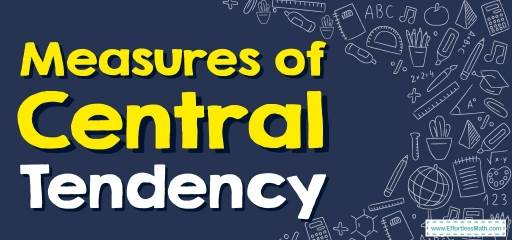# How to Find the Measures of Central Tendency? (+FREE Worksheet!)In this article, you will learn how to find the Measures of Central Tendency in a few simple steps.

## Step by step guide to Find the Measures of Central Tendency

Measures of Variability allow us to summarize an entire data set with a single value. Mean, Mode, and Median are all three examples of Measures of Central Tendency.

Mean: It means average, and to calculate mean, we divide the sum of the numbers by the number of observations. Can be used with discrete and continuous data.

Median: The middle value that separates the higher half from the lower half. Numbers are arranged in either ascending or descending order. The middle number is then taken.

Mode: the most frequent value. It uses to show the most popular option and is the highest bar in the histogram.

To decide which one to use, the following should be considered:

• The mean gives all values the same importance, even very large or very small values, while the median focuses more on the values that are in the middle of the data set; So, the mean can use the data more fully. However, as mentioned, the mean can be strongly influenced by one or two very large or very small values.
• There is always only one value for the median or mean on a data set, but a set can have more than one mode.
• Among the Measures of Central Tendency, the mode is less used than the mean and median. However, in some cases, the mode can be significantly useful.

### Finding the Measures of Central Tendency Example 1:

Find the mean, median, and mode of the data set. $$10, 11, 25, 16, 16, 46, 29, 35$$

Solution: Mean: first add the values $$10+11+25+16+16+46+29+35=188$$

Divide by $$8$$, the number of values. Mean$$=\frac{188}{8}=23.5$$

Median: order the data from least to greatest. $$10, 11, 16, 16, 25, 29, 35, 45$$

Average the two middle values. $$=\frac{16+25}{2}=20.5$$

So, $$20.5$$ is median.

Mode: the value $$16$$ occurs two times. So, $$16$$ is the mode.

### Finding the Measures of Central Tendency Example 2:

Find the mean, median, and mode of the data set. $$22, 35, 45, 53, 52, 35, 11, 16, 35, 11$$

Solution: Mean: first add the values $$22+35+45+53+52+35+11+16+35+11=315$$

Divide by $$10$$, the number of values. Mean$$=\frac{315}{10}=31.5$$

Median: order the data from least to greatest. $$11, 11, 16, 22, 35, 35, 35, 45, 52, 53$$

Average the two middle values. $$=\frac{35+35}{2}=35$$

So, $$35$$ is median.

Mode: the value $$35$$ occurs three times. So, $$35$$ is the mode.

### Finding the Measures of Central Tendency Example 3:

Find the mean, median, and mode of the data set. $$9, 2, 5, 21, 16, 5, 36, 13, 10$$

Solution: Mean: first add the values $$9+2+5+21+16+5+36+13+10=117$$

Divide by $$9$$, the number of values. Mean$$=\frac{117}{9}=13$$

Median: order the data from least to greatest. $$2, 5, 5, 9, 10, 13, 16, 21, 36$$

The middle value is the median. So, median$$=10$$

Mode: the value $$5$$ occurs two times. So, $$5$$ is the mode.

### Finding the Measures of Central Tendency Example 4:

Find the mean, median, and mode of the data set. $$25, 36, 39, 8, 17, 45, 60, 1, 36, 42, 10$$

Solution: Mean: first add the values $$25+36+39+8+17+45+60+1+36+42+10=319$$

Divide by $$11$$, the number of values. Mean$$=\frac{319}{11}=29$$

Median: order the data from least to greatest. $$1, 8, 10, 17,25, 36, 36, 39, 42, 45, 60$$

The middle value is the median. So, median$$=36$$

Mode: the value $$36$$ occurs two times. So, $$36$$ is the mode.

## Exercises for Finding the Measures of Central Tendency

### Calculate the mean, median, and mode for the following data sets.

1. Points scored by a basketball player: {$$8, 3, 17, 26, 13, 3, 30$$}
2. Marks on a set of tests: {$$64, 88, 95, 75, 69, 88, 70, 77$$}
3. Waiting time, in minutes: {$$13, 19, 11, 19, 7, 32, 45, 33$$}
4. Monthly rent : {$$630, 585, 670, 710, 670, 600, 590$$}
1. Mean: $$14.29$$ Median: $$13$$ Mode: $$3$$
2. Mean: $$78.25$$ Median: $$76$$ Mode: $$88$$
3. Mean: $$22.38$$ Median: $$19$$ Mode: $$19$$
4. Mean: $$636.43$$ Median: $$630$$ Mode: $$670$$

### What people say about "How to Find the Measures of Central Tendency? (+FREE Worksheet!) - Effortless Math: We Help Students Learn to LOVE Mathematics"?

No one replied yet.

X
30% OFF

Limited time only!

Save Over 30%

SAVE $5 It was$16.99 now it is \$11.99